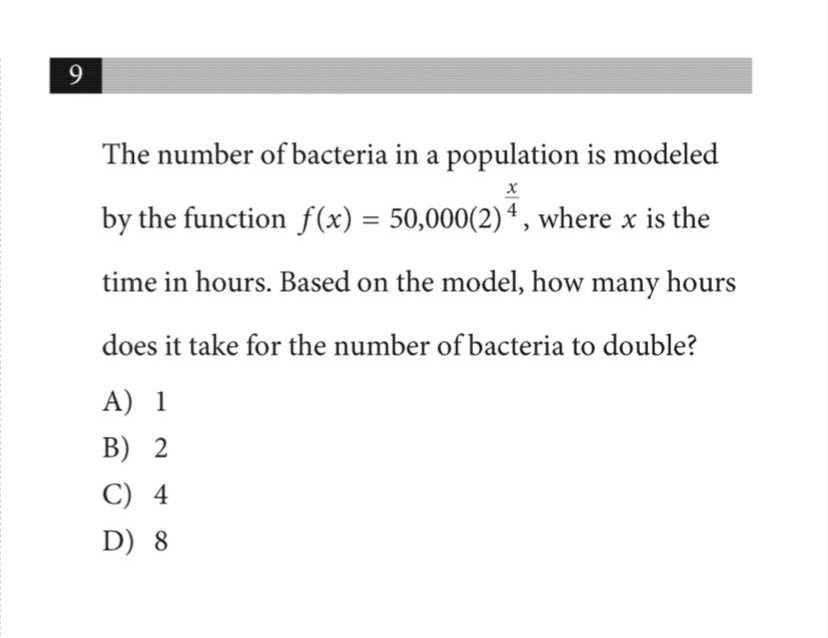### ¿Todavía tienes preguntas de matemáticas?

Pregunte a nuestros tutores expertos
Algebra
Pregunta9 The number of bacteria in a population is modeled by the function $$f ( x ) = 50,000 ( 2 ) ^ { \frac { x } { 4 } }$$ , where $$x$$ is the time in hours. Based on the model, how many hours does it take for the number of bacteria to double?

A) $$1$$

B) $$2$$

C) $$4$$

D) $$8$$